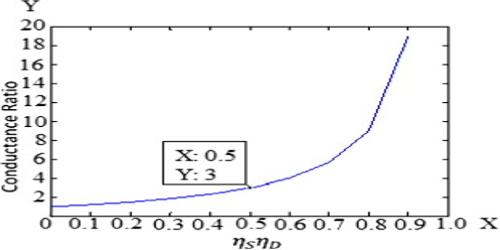# The Conductance Ratio

The Conductance Ratio

The conductance of one mole of a particular electrolyte in solution will depend on (a) the number of ions and (b) the speeds of the ions. The difference in the molar conductance of different electrolytes may he attributed to difference in speeds. The variation of molar conductance of a given electrolyte with concentration was explained by Arrhenius by assuming that the extent of dissociation of the electrolyte increases with increasing dilution. At infinite dilution the dissociation would be complete and the value of A would not change with further dilution, i.e., the molar conductance would reach its limiting value, Λ0. Since the molar conductance at any concentration, Λc, is proportional to the number of ions, the extent of dissociation at the concentration, c, would be given by,

α = Λc0

Arrhenius named this quantity the degree of dissociation. As will be seen in “Conductance and Electrolyte Concentration”, all strong electrolytes are now supposed to be completely dissociated into ions in solution, and the term has little significance except in the case of weak electrolytes which are regarded as incompletely dissociated. The ratio (Λc0) is now given the non-committal term, conductance ratio.